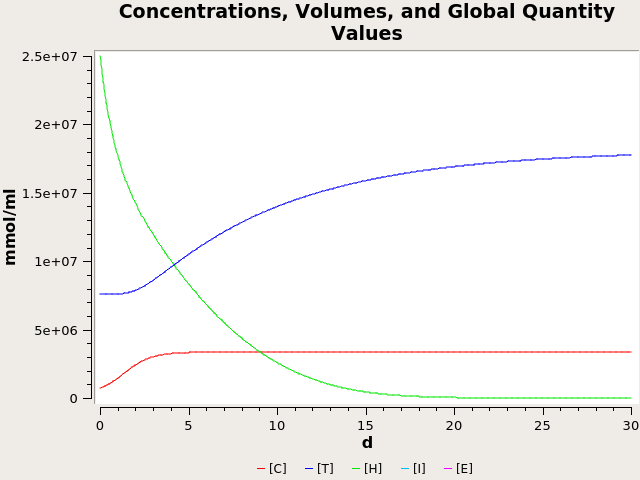## Solis-perez2019 - A fractional mathematical model of breast cancer competition modelModel Identifier
BIOMD0000000903
Short description
A fractional mathematical model of breast cancer competition model
bA.Atanganac

a
Tecnológico Nacional de México/CENIDET. Interior Internado Palmira S/N, Col. Palmira, C.P. 62490, Cuernavaca, Morelos, México

b
CONACyT-Tecnológico Nacional de México/CENIDET. Interior Internado Palmira S/N, Col. Palmira, C.P. 62490, Cuernavaca, Morelos, México

c
Institute for Groundwater Studies, Faculty of Natural and Agricultural Sciences, University of the Free State, Bloemfontein 9300, South Africa

Abstract

In this paper, a mathematical model which considers population dynamics among cancer stem cells, tumor cells, healthy cells, the effects of excess estrogen and the body’s natural immune response on the cell populations was considered. Fractional derivatives with power law and exponential decay law in Liouville–Caputo sense were considered. Special solutions using an iterative scheme via Laplace transform were obtained. Furthermore, numerical simulations of the model considering both derivatives were obtained using the Atangana–Toufik numerical method. Also, random model described by a system of random differential equations was presented. The use of fractional derivatives provides more useful information about the complexity of the dynamics of the breast cancer competition model.
Format
SBML (L2V4)
Related Publication
• A fractional mathematical model of breast cancer competition model• J.E.Solís-Pérez a J.F.Gómez-Aguilar b A.Atangana c
• Chaos, Solitons & Fractals , 10/ 2019 , Volume 127 , pages: 38-54 , DOI: 10.1016/j.chaos.2019.06.027
• a Tecnológico Nacional de México/CENIDET. Interior Internado Palmira S/N, Col. Palmira, C.P. 62490, Cuernavaca, Morelos, México b CONACyT-Tecnológico Nacional de México/CENIDET. Interior Internado Palmira S/N, Col. Palmira, C.P. 62490, Cuernavaca, Morelos, México c Institute for Groundwater Studies, Faculty of Natural and Agricultural Sciences, University of the Free State, Bloemfontein 9300, South Africa
• In this paper, a mathematical model which considers population dynamics among cancer stem cells, tumor cells, healthy cells, the effects of excess estrogen and the body’s natural immune response on the cell populations was considered. Fractional derivatives with power law and exponential decay law in Liouville–Caputo sense were considered. Special solutions using an iterative scheme via Laplace transform were obtained. Furthermore, numerical simulations of the model considering both derivatives were obtained using the Atangana–Toufik numerical method. Also, random model described by a system of random differential equations was presented. The use of fractional derivatives provides more useful information about the complexity of the dynamics of the breast cancer competition model. Volume 127, October 2019, Pages 38-54
Contributors
Submitter of the first revision: Mohammad Umer Sharif Shohan
Submitter of this revision: Mohammad Umer Sharif Shohan

is (2 statements)
BioModels Database MODEL1912180005
BioModels Database BIOMD0000000903

hasInstance (3 statements)
Taxonomy Homo sapiens
Mathematical Modelling Ontology Ordinary differential equation model
NCIt Breast Carcinoma

hasTaxon (1 statement)
Taxonomy Homo sapiens

hasProperty (1 statement)
Mathematical Modelling Ontology Ordinary differential equation model

isDescribedBy (2 statements)
isDerivedFrom (1 statement)

Curation status
Curated

Modelling approach(es)

Tags

#### Connected external resources

SBGN view in Newt Editor

Name Description Size Actions

### Model files

Solis-perez2019.xml SBML L2V4 representation of Solis-perez2019 - A fractional mathematical model of breast cancer competition model 53.56 KB Preview | Download

Solis-perez2019.cps COPASI version 4.24 (Build 197) Solis-perez2019 - A fractional mathematical model of breast cancer competition model 102.41 KB Preview | Download
Solis-perez2019.sedml SEDML L1V2 Solis-perez2019 - A fractional mathematical model of breast cancer competition model 3.61 KB Preview | Download
• Model originally submitted by : Mohammad Umer Sharif Shohan
• Submitted: Dec 18, 2019 2:41:48 PM
##### Revisions
Legends
: Variable used inside SBML models

Species
Species Initial Concentration/Amount
C

BTO:0006293
737100.0 mmol
T

Neoplastic Cell
7616700.0 mmol
E 0.0 mmol
I

Immune Cell
0.0 mmol
H

Healthy
2.5E7 mmol
Reactions
Reactions Rate Parameters
=> C; E compartment*(k1*C*(1-C/M1)+p1*C*E/(a1+C)) p1 = 600.0; M1 = 2270000.0; k1 = 0.75; a1 = 1135000.0
T => ; I compartment*(n1*T+gamma2*I*T) gamma2 = 3.0E-6; n1 = 0.01
=> E compartment*tau tau = 2700.0
E => ; C, T, H compartment*(mu+d1*C/(a1+C)+d2*T/(a2+T)+d3*H/(a3+H))*E a2 = 1.135E7; mu = 0.97; a3 = 1250000.0; a1 = 1135000.0; d1 = 0.01; d2 = 0.01; d3 = 0.01
I => ; T, E compartment*(gamma3*I*T+n2*I+u*I*E/(v+E)) gamma3 = 1.0E-7; v = 400.0; u = 0.2; n2 = 0.29
=> I; T compartment*(s+p*I*T/(w+T)) s = 13000.0; w = 300000.0; p = 0.2
C => ; I compartment*gamma1*I*C gamma1 = 3.0E-7
H => ; T, E compartment*(delta*H*T+p3*H*E/(a3+H)) a3 = 1250000.0; p3 = 100.0; delta = 6.0E-8
=> H compartment*q*H*(1-H/M3) q = 0.7; M3 = 2.5E7
=> T; C, E compartment*(k2*C*C/M1*(1-T/M2)+p2*T*E/(a2+T)) p2 = 0.0; M2 = 2.27E7; a2 = 1.135E7; M1 = 2270000.0; k2 = 0.514Curator's comment:
(added: 18 Dec 2019, 14:41:36, updated: 18 Dec 2019, 14:41:36)
The model has been encode in COPASI 4.24 (Build 197) and the Figure 1 of the publication has been reproduced using COPASI